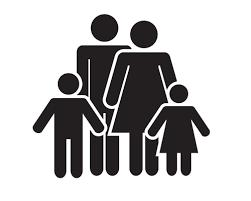# Family

Mom is 42 years old, and her daughters are 13 and 19. After how many years will a mother become as old as her daughter together?

x =  10

### Step-by-step explanation:

42 + x = (13+x)+ (19+x)

x = 10

x = 10/1 = 10

x = 10

Our simple equation calculator calculates it.Did you find an error or inaccuracy? Feel free to write us. Thank you!

Tips for related online calculators
Do you have a linear equation or system of equations and looking for its solution? Or do you have a quadratic equation?
Do you want to convert time units like minutes to seconds?